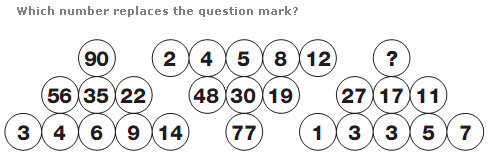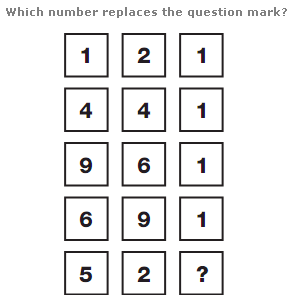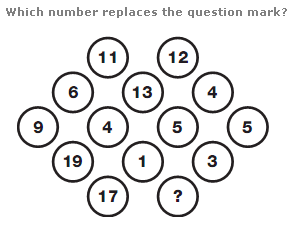# Puzzles - Number puzzles

### Exercise :: Number puzzlesAnswer : 43 Explanation : In each triangle shape, start at the left hand side of the longest row and move to the right, then on the next row and to the left, and finally to the third row. Add together the first two numbers and subtract 1 to give the next value around.Answer : 2 Explanation : Working from top to bottom, and reading each row as a 3 digit number, rows are arranged in order of the Square Numbers, from 11 to 15, with the digits reversed in each case.Answer : 6 Explanation : Work through the diagram in horizontal rows. The sum of the numbers in each row is always 23.